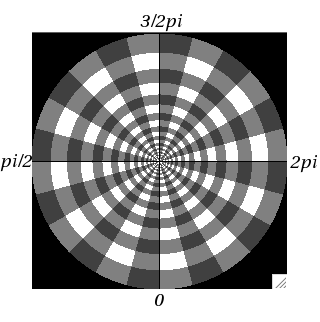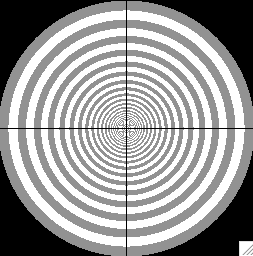## Sunday, March 25, 2007

### Matlab and huge matrix execution time

Recently I was working with huge, really huge 3D matrixes in Matlab for example take matrix `A=ones(64,64,50100)`. It is huge matrix of double values. Unfortunately operations on such a matrix are very time consuming.

For example performing summation of all values along the third dimension `sum(A,3)`
takes 235 seconds on Ultra Sparc Sun-Fire-440. That is why sometimes it maybe beneficial to create single or unsigned integer 32bit (uint32) matrix instead of doubles e.g: `A=uint32(ones(64,64,50100))`. In this case performing sum over third dimension takes 211 seconds. When using single instead of double, the time is 204 sec. The difference is not so big, however when using uint8 the time is only 60s. Unfortunately using uint8 when one wants to sum up matrix with over 50000 numbers has no sense unless it is heavily sparse matrix - uint8 holds numbers not bigger than 256.

In conclusion, I think it is better to avoid such matrixes.

## Wednesday, March 21, 2007

### Directional evaluation of fractal dimension using Power Spectrum Method

Power spectrum algorithm, based on J. Russ book  divided 2D power spectrum of an imput image into 'slices' which represent directions and frequencies as show below:For example one can divide power spectrum i to 24 radial slices i.e. one slice is 15 degrees (24*15=360) and slice is divided to 30 frequencies (30 rings). The greater radius of the ring, the bigger frequency.
Apart from directional fractal dimension evaluation, algorithm performs overall fractal dimension analysis by 'averaging by circles'. In this case power spectrum is divided to 60 (2*30) rings:In addition, vertical and horizontal direction are not considered in the computation as to avoid border effects.

John C. Russ, Fractal Surfaces, 1994